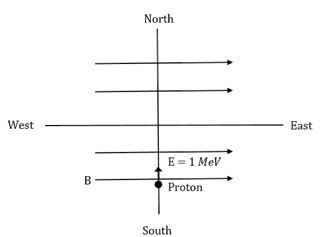Proton with kinetic energy of 1 MeV moves from south to north...

Proton with kinetic energy of 1 MeV moves from south to north. It gets an acceleration of 1012 m/s2 by an applied magnetic field (west to east). The value of magnetic field: (Rest mass of proton is 1.6 × 10−27 kg)a. 0.71 mT

b. 7.1 mT

c. 71 mT

d. 0.071 mT

Solution:

K. E = 1 × 106 eV = 1.6 × 10−13 J

= ½ mev2

Where me is the mass of the electron = 1.6×10 -27

1.6 × 10-13 = ½ × 1.6 × 10-27 × v2

v = √2×107 m/s

Bqv = mea

B = (1.6 ×10 -27 × 1012 )/ (1.6×10-19×√2× 107)

= 0.71 × 10-3 T

= 0.71 mT(0)(0)